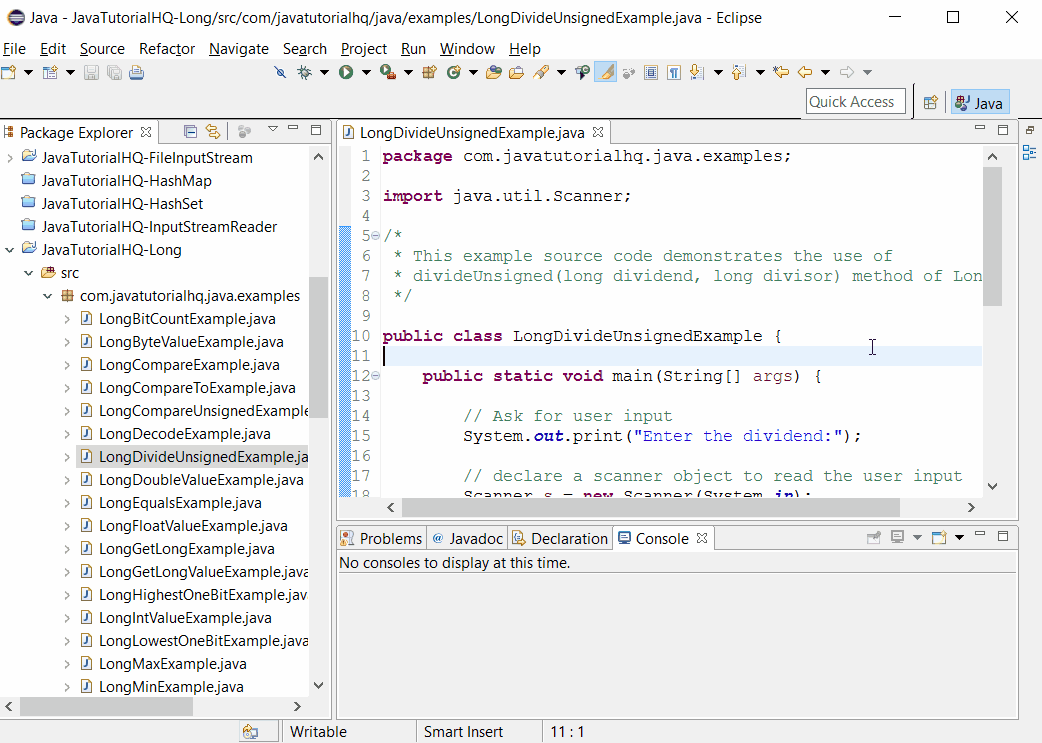# Java long divideUnsigned() method example

HQ » Java Tutorial » java.lang » Long » divideUnsigned(long dividend, long divisor)
Java long divideUnsigned() method example 2018-02-16T07:26:14+00:00

## Description

The Long.divideUnsigned(long dividend, long divisor) java method returns the unsigned quotient of dividing the first argument by the second where each argument and the result is interpreted as an unsigned value.

Note that in two’s complement arithmetic, the three other basic arithmetic operations of add, subtract, and multiply are bit-wise identical if the two operands are regarded as both being signed or both being unsigned. Therefore separate addUnsigned, etc. methods are not provided.

Make a note that the divideUnsigned() method of Long class is static thus it should be accessed statically which means the we would be calling this method in this format:

Long.divideUnsigned(method args)

Non static method is usually called by just declaring method_name(argument) however in this case since the method is static, it should be called by appending the class name as suffix. We will be encountering a compilation problem if we call the java divideUnsigned method non statically.

## Method Syntax

public static long divideUnsigned(long dividend, long divisor)

## Method Argument

Data Type Parameter Description
long dividend the value to be divided
long divisor the value doing the dividing

## Method Returns

The divideUnsigned(long dividend, long divisor) method of Long class returns the unsigned quotient of the first argument divided by the second argument

## Compatibility

Requires Java 1.8 and up

## Java Long divideUnsigned() Example

Below is a simple java example on the usage of divideUnsigned() method of Long class.

## Sample Output

Below is the sample output when you run the above example.## References

Singapore

#### Our Goal

JavaTutorialHQ aims to to be The Ultimate Guide on Java with hundreds of examples from basic to advance Topics.

By continuing to use the site, you agree to the use of cookies. more information

The cookie settings on this website are set to "allow cookies" to give you the best browsing experience possible. If you continue to use this website without changing your cookie settings or you click "Accept" below then you are consenting to this.

Close